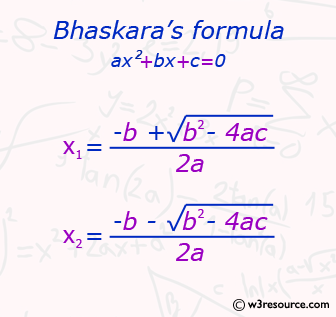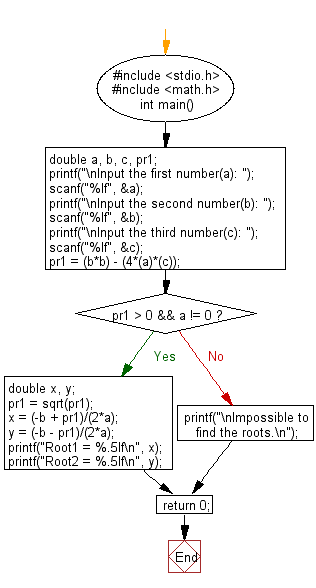﻿ C : Roots of Bhaskaras formula based on 3 floating numbers

# C Exercises: Print the roots of Bhaskara’s formula from the given three floating numbers

## C Basic Declarations and Expressions: Exercise-20 with Solution

Write a C program to print the roots of Bhaskara’s formula from the given three floating numbers. Display a message if it is not possible to find the roots.

Pictorial Presentation:C Code:

``````#include <stdio.h>
#include <math.h>
// Include math.h header file for mathematical functions

int main() {
double a, b, c, pr1; // Declare variables for coefficients and discriminant

// Prompt user for coefficients 'a', 'b', and 'c'
printf("\nInput the first number(a): ");
scanf("%lf", &a);
printf("\nInput the second number(b): ");
scanf("%lf", &b);
printf("\nInput the third number(c): ");
scanf("%lf", &c);

pr1 = (b*b) - (4*(a)*(c)); // Calculate discriminant

if(pr1 > 0 && a != 0) { // Check conditions for real roots
double x, y;
pr1 = sqrt(pr1); // Calculate square root of discriminant
x = (-b + pr1)/(2*a); // Calculate first root
y = (-b - pr1)/(2*a); // Calculate second root
printf("Root1 = %.5lf\n", x); // Print first root
printf("Root2 = %.5lf\n", y); // Print second root
}
else {
printf("\nImpossible to find the roots.\n"); // Print message for no real roots
}

return 0;
}
``````

Sample Output:

```Input the first number(a): 25

Input the second number(b): 35

Input the third number(c): 12
Root1 = -0.60000
Root2 = -0.80000
```

Flowchart:C Programming Code Editor:

What is the difficulty level of this exercise?

Test your Programming skills with w3resource's quiz.

﻿